# 双线性插值 Bilinear Interpolation机器视觉 同时被 2 个专栏收录2 篇文章 0 订阅

## Definition

1. In mathematics, bilinear interpolation is an extension of linear interpolation for interpolating functions of two variables (e.g. x and y) on a rectilinear 2D grid.
2. Bilinear interpolation is performed using linear interpolation first in one direction and then again in the other direction. Although each step is linear in the sampled values and in the position, the interpolation as a whole is not linear but rather quadratic in the sample location.
3. It’s one of the basic resampling techniques in computer vision and image processing, where it is also called bilinear filtering or bilinear texture mapping.
4. red: data points; green: point at which we want to interpolate.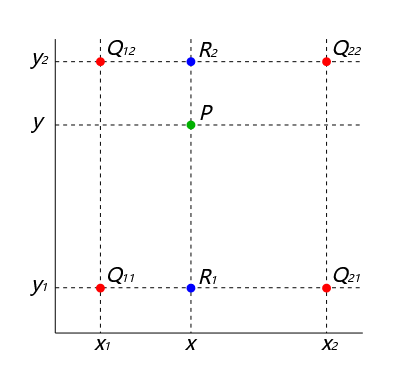5. A geometric visualisation of bilinear interpolation. The product of the value at the desired point (black) and the entire area is equal to the sum of the products of the value at each corner and the partial area diagonally opposite the corner (corresponding colours).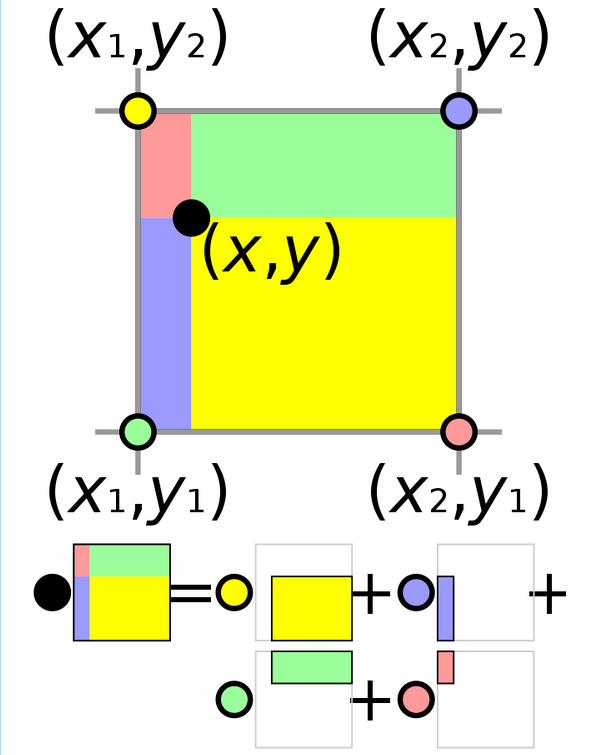## Algorithm

Suppose we want to find the value of the unknown function f at the point (x,y). It is assumed that we know the value of f f at the four points Q 11 = ( x 1 , y 1 ) , Q 12 = ( x 1 , y 2 ) , Q 21 = ( x 2 , y 1 ) , Q 22 = ( x 2 , y 2 ) . Q_{11} = (x_1, y_1), Q_{12} = (x_1, y_2), Q_{21} = (x_2, y_1), Q_{22} = (x_2, y_2).

We first do linear interpolation in the x x -direction. This yields:

f ( x , y 1 ) ≈ x 2 − x x 2 − x 1 f ( Q 11 ) + x − x 1 x 2 − x 1 f ( Q 21 ) f(x,y_1)\approx \frac{x_2-x}{x_2-x_1} f(Q_{11}) + \frac{x-x_1}{x_2-x_1}f(Q_{21})

f ( x , y 2 ) ≈ x 2 − x x 2 − x 1 f ( Q 12 ) + x − x 1 x 2 − x 1 f ( Q 22 ) f(x,y_2)\approx \frac{x_2-x}{x_2-x_1} f(Q_{12}) + \frac{x-x_1}{x_2-x_1}f(Q_{22})

We proceed by interpolating in the y y -direction to obtain the desired estimate: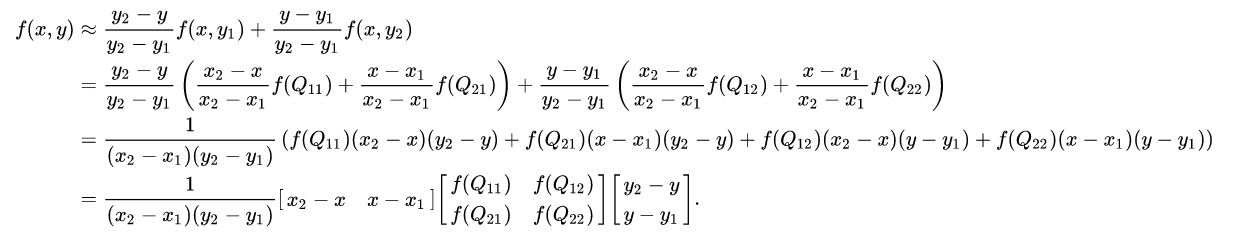### Alternative algorithm

An alternative way to write the solution to the interpolation problem is

f ( x , y ) ≈ a 0 + a 1 x + a 2 y + a 3 x y f(x,y) \approx a_0 + a_1x+a_2y+a_3xy

where the coefficients are found by solving the linear system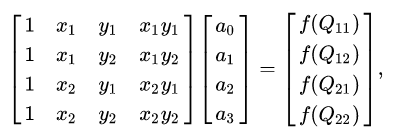yielding the result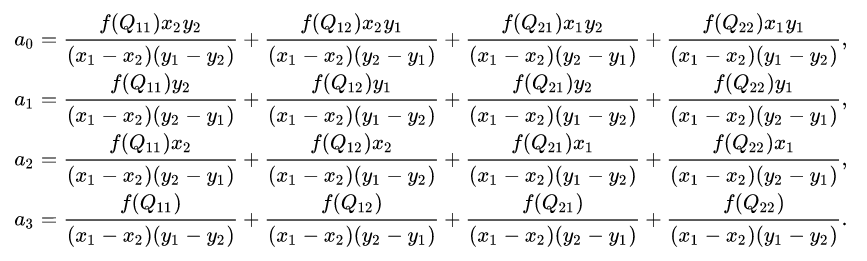### Unit square

If we choose a coordinate system in which the four points where f f is know are ( 0 , 0 ) , ( 1 , 0 ) , ( 0 , 1 ) , ( 1 , 1 ) (0,0), (1,0), (0,1), (1,1) , then the interpolation formula simplifies to

f ( x , y ) ≈ f ( 0 , 0 ) ( 1 − x ) ( 1 − y ) + f ( 1 , 0 ) x ( 1 − y ) + f ( 0 , 1 ) ( 1 − x ) y + f ( 1 , 1 ) x y f(x,y) \approx f(0,0)(1-x)(1-y)+f(1,0)x(1-y)+f(0,1)(1-x)y+f(1,1)xy

or equivalently, in matrix operations: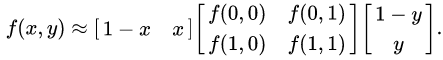### Nonlinear

As the name suggests, the bilinear interpolant is not linear, but the product of two linear functions. For example, the bilinear interpolation derived above is a product of the values of x x and y y .

Alternatively, the interpolant on the unit square can be written as

f ( x , y ) = ∑ i = 1 2 ∑ j = 1 2 a i j x i − 1 y i − 1 = a 11 + a 21 x + a 12 y + a 22 x y f(x,y) = \sum_{i=1}^2\sum_{j=1}^2a_{ij}x^{i-1}y^{i-1}=a_{11}+a_{21}x+a_{12}y+a_{22}xy

where

a 11 = f ( 1 , 1 ) a_{11}=f(1,1)
a 21 = f ( 2 , 1 ) − f ( 1 , 1 ) a_{21}=f(2,1)-f(1,1)
a 12 = f ( 1 , 2 ) − f ( 1 , 1 ) a_{12}=f(1,2)-f(1,1)
a 22 = f ( 2 , 2 ) + f ( 1 , 1 ) − ( f ( 2 , 1 ) + f ( 1 , 2 ) ) a_{22}=f(2,2)+f(1,1)-\left(f(2,1)+f(1,2)\right)

In both cases, the number of constants (four) correspond to the number of data points where f f is given. The interpolant is linear along lines parallel to either the x or the y direction, equivalently if x x or y y is set constant. Along any other straight line, the interpolant is quadratic. However, even if the interpolation is not linear in the position (x and y), it is linear in the amplitude, as it is apparent from the equations above: all the coefficients a j , j ∈ { 1 , 2 , 3 , 4 } a_j, j \in \{1,2,3,4\} are proportional to the value of the function f f .

The result of bilinear interpolation is independent of which axis is interpolated first and which second. If we had first performed the linear interpolation in the y direction and then in the x direction, the resulting approximation would be the same.

The obvious extension of bilinear interpolation to three dimensions is called trilinear interpolation.

## Application in image processing

In computer vision and image processing, bilinear interpolation is used to resample images and textures. An algorithm is used to map a screen pixel location to a corresponding point on the texture map. A weighted average of the attributes (color, transparency, etc.) of the four surrounding texels is computed and applied to the screen pixel. This process is repeated for each pixel forming the object being textured.

When an image needs to be scaled up, each pixel of the original image needs to be moved in a certain direction based on the scale constant. However, when scaling up an image by a non-integral scale factor, there are pixels (i.e., holes) that are not assigned appropriate pixel values. In this case, those holes should be assigned appropriate RGB or grayscale values so that the output image does not have non-valued pixels.

Bilinear interpolation can be used where perfect image transformation with pixel matching is impossible, so that one can calculate and assign appropriate intensity values to pixels. Unlike other interpolation techniques such as nearest-neighbor interpolation and bicubic interpolation, bilinear interpolation uses values of only the 4 nearest pixels, located in diagonal directions from a given pixel, in order to find the appropriate color intensity values of that pixel.

Bilinear interpolation considers the closest 2 × 2 neighborhood of known pixel values surrounding the unknown pixel’s computed location. It then takes a weighted average of these 4 pixels to arrive at its final, interpolated value.

Texel: In computer graphics, a texel, texture element, or texture pixel is the fundamental unit of a texture map. Textures are represented by arrays of texels representing the texture space, just as other images are represented by arrays of pixels.

### Example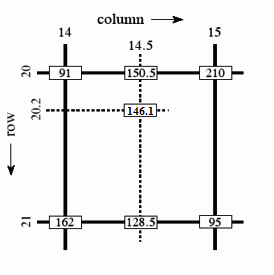As shown in the image above, the intensity value at the pixel computed to be at row 20.2, column 14.5 can be calculated by first linearly interpolating between the values at column 14 and 15 on each rows 20 and 21, giving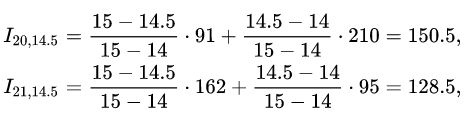and then the interpolating linearly between these values, giving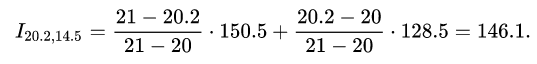This algorithm reduces some of the visual distortion caused by resizing an image to a non-integral zoom factor, as opposed to nearest-neighbor interpolation, which will make some pixels appear larger than others in the resized image.

## Reference

03-21102302-02709
12-12708
12-01336
05-074万+
11-27436
04-227万+
03-253161
04-111725
12-042626
10-135994
03-29487
12-143176
03-121万+
12-199294
10-18点击重新获取扫码支付余额充值